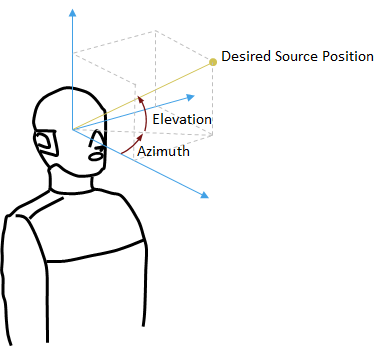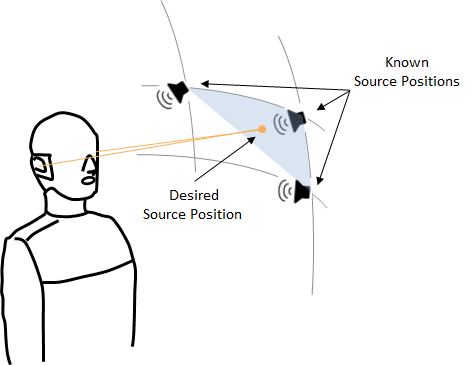Documentation

# interpolateHRTF

3-D head-related transfer function (HRTF) interpolation

## Syntax

``interpolatedHRTF = interpolateHRTF(HRTF,sourcePositions,desiredSourcePositions)``
``interpolatedHRTF = interpolateHRTF(___,Name,Value)``

## Description

example

````interpolatedHRTF = interpolateHRTF(HRTF,sourcePositions,desiredSourcePositions)` returns the interpolated head-related transfer function (HRTF) at the desired position.```

example

````interpolatedHRTF = interpolateHRTF(___,Name,Value)` specifies options using one or more `Name,Value` pair arguments.```

## Examples

collapse all

Modify the 3-D audio image of a sound file by filtering it through a head-related transfer function (HRTF). Set the location of the sound source by specifying the desired azimuth and elevation.

```load 'ReferenceHRTF.mat' hrtfData sourcePosition hrtfData = permute(double(hrtfData),[2,3,1]); sourcePosition = sourcePosition(:,[1,2]);```

Calculate the head-related impulse response (HRIR) using the VBAP algorithm at a desired source position. Separate the output, `interpolatedIR`, into the impulse responses for the left and right ears.

```desiredAz = 110; desiredEl = -45; desiredPosition = [desiredAz desiredEl]; interpolatedIR = interpolateHRTF(hrtfData,sourcePosition,desiredPosition, ... "Algorithm","VBAP"); leftIR = squeeze(interpolatedIR(:,1,:))'; rightIR = squeeze(interpolatedIR(:,2,:))';```

Create a `dsp.AudioFileReader` object to read in a file frame by frame. Create an `audioDeviceWriter` object to play audio to your sound card frame by frame. Create two `dsp.FIRFilter` objects and specify the filter coefficients using the head-related transfer function interpolated impulse responses.

```fileReader = dsp.AudioFileReader('RockDrums-44p1-stereo-11secs.mp3'); deviceWriter = audioDeviceWriter('SampleRate',fileReader.SampleRate); leftFilter = dsp.FIRFilter('Numerator',leftIR); rightFilter = dsp.FIRFilter('Numerator',rightIR);```

In an audio stream loop:

1. Read in a frame of audio data.

2. Feed the stereo audio data through the left and right HRIR filters, respectively.

3. Concatenate the left and right channels and write the audio to your output device.

```while ~isDone(fileReader) audioIn = fileReader(); leftChannel = leftFilter(audioIn(:,1)); rightChannel = rightFilter(audioIn(:,2)); deviceWriter([leftChannel,rightChannel]); end```

As a best practice, release your System objects when complete.

```release(deviceWriter) release(fileReader)```

Create arrays of head-related impulse responses corresponding to desired source positions. Filter mono input to model a moving source.

Load the ARI HRTF dataset. Cast the `hrtfData` to type double, and reshape it to the required dimensions: (number of source positions)-by-2-by-(number of HRTF samples). Use the first two columns of the `sourcePosition` matrix only, which correspond to the azimuth and elevation of the source in degrees.

```load 'ReferenceHRTF.mat' hrtfData sourcePosition hrtfData = permute(double(hrtfData),[2,3,1]); sourcePosition = sourcePosition(:,[1,2]);```

Specify the desired source positions and then calculate the HRTF at these locations using the `interpolateHRTF` function. Separate the output, `interpolatedIR`, into the impulse responses for the left and right ears.

```desiredAz = [-120;-60;0;60;120;0;-120;120]; desiredEl = [-90;90;45;0;-45;0;45;45]; desiredPosition = [desiredAz desiredEl]; interpolatedIR = interpolateHRTF(hrtfData,sourcePosition,desiredPosition); leftIR = squeeze(interpolatedIR(:,1,:)); rightIR = squeeze(interpolatedIR(:,2,:));```

Create a `dsp.AudioFileReader` object to read in a file frame by frame. Create an `audioDeviceWriter` object to play audio to your sound card frame by frame. Create two `dsp.FIRFilter` objects with `NumeratorSource` set to `Input port`. Setting `NumeratorSource` to `Input port` enables you to modify the filter coefficients while streaming.

```leftFilter = dsp.FIRFilter('NumeratorSource','Input port'); rightFilter = dsp.FIRFilter('NumeratorSource','Input port'); fileReader = dsp.AudioFileReader('Counting-16-44p1-mono-15secs.wav'); deviceWriter = audioDeviceWriter('SampleRate',fileReader.SampleRate);```

In an audio stream loop:

1. Read in a frame of audio data.

2. Feed the audio data through the left and right HRIR filters.

3. Concatenate the left and right channels and write the audio to your output device. If you have a stereo output hardware, such as headphones, you can hear the source shifting position over time.

4. Modify the desired source position in 2-second intervals by updating the filter coefficients.

```durationPerPosition = 2; samplesPerPosition = durationPerPosition*fileReader.SampleRate; samplesPerPosition = samplesPerPosition - rem(samplesPerPosition,fileReader.SamplesPerFrame); sourcePositionIndex = 1; samplesRead = 0; while ~isDone(fileReader) audioIn = fileReader(); samplesRead = samplesRead + fileReader.SamplesPerFrame; leftChannel = leftFilter(audioIn,leftIR(sourcePositionIndex,:)); rightChannel = rightFilter(audioIn,rightIR(sourcePositionIndex,:)); deviceWriter([leftChannel,rightChannel]); if mod(samplesRead,samplesPerPosition) == 0 sourcePositionIndex = sourcePositionIndex + 1; end end```

As a best practice, release your System objects when complete.

```release(deviceWriter) release(fileReader)```

## Input Arguments

collapse all

HRTF values measured at the source positions, specified as a N-by-2-by-M array.

• N –– Number of known HRTF pairs

• M –– Number of samples in each known HRTFIf you specify `HRTF` with real numbers, the function assumes that the input represents an impulse response, and M corresponds to the length of the impulse response. If you specify `HRTF` with complex numbers, the function assumes that the input represents a transfer function, and M corresponds to the number of bins in the frequency response. The output of the `interpolateHRTF` function has the same complexity and interpretation as the input.

Data Types: `single` | `double`
Complex Number Support: Yes

Source positions corresponding to measured HRTF values, specified as a N-by-2 matrix. N is the number of known HRTF pairs. The two columns correspond to the azimuth and elevation of the source in degrees, respectively.

Azimuth must be in the range [−180,360]. You can use the −180 to 180 convention or the 0 to 360 convention.

Elevation must be in the range [−90,180]. You can use the −90 to 90 convention or the 0 to 180 convention.

Data Types: `single` | `double`

Desired source position for HRTF interpolation, specified as a P-by-2 matrix. P is the number of desired source positions. The columns correspond to the desired azimuth and elevation of the source in degrees, respectively.

Azimuth must be in the range [−180,360]. You can use the −180 to 180 convention or the 0 to 360 convention.

Elevation must be in the range [−90,180]. You can use the −90 to 90 convention or the 0 to 180 convention.Data Types: `single` | `double`

### Name-Value Pair Arguments

Specify optional comma-separated pairs of `Name,Value` arguments. `Name` is the argument name and `Value` is the corresponding value. `Name` must appear inside quotes. You can specify several name and value pair arguments in any order as `Name1,Value1,...,NameN,ValueN`.

Example: `'Algorithm','VBAP'`

Interpolation algorithm, specified as `"Bilinear"` or `"VBAP"`.

• `Bilinear` –– 3-D bilinear interpolation, as specified by .

• `VBAP` –– Vector base amplitude panning interpolation, as specified by .

Data Types: `char` | `string`

## Output Arguments

collapse all

Interpolated HRTF, returned as a P-by-2-by-M array.`interpolatedHRTF` has the same complexity and interpretation as the input. If you specify the input, `HRTF`, with real numbers, the function assumes that the input represents an impulse response. If you specify the input with complex numbers, the function assumes that the input represents a transfer function.

Data Types: `single` | `double`
Complex Number Support: Yes

 F.P. Freeland, L.W.P. Biscainho and P.S.R. Diniz, "Interpolation of Head-Related Transfer Functions (HRTFS): A multi-source approach." 2004 12th European Signal Processing Conference. Vienna, 2004, pp. 1761–1764.

 Pulkki, Ville. "Virtual Sound Source Positing Using Vector Base Amplitude Panning." Journal of Audio Engineering Society. Vol. 45. Issue 6, pp. 456–466.

##### Support